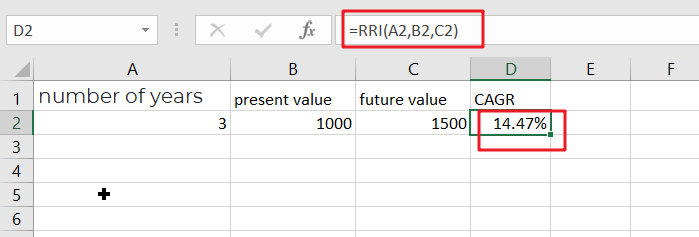# How To Use Excel RRI Function

This post will guide you how to use Excel RRI function with syntax and examples in Microsoft excel.

## Description

The Excel RRI function Returns an equivalent interest rate for the growth of an investment. So you can use the RRI function to get the the growth of an investment based on present and future value of the investment.

The RRI function is a build-in function in Microsoft Excel and it is categorized as a Financial Function.

The RRI function is available in Excel 2016, Excel 2013, Excel 2010, Excel 2007, Excel 2011 for Mac.

## Syntax

The syntax of the RRI function is as below:

`= RRI (nper,pv,fv)`

Where the PV function arguments are:

• nPer -This is a required argument. The total number of periods for the investment.
• pv – This is required argument. The present value is the future value of the investment
• fv – This is required argument. The future value is the future value of the investment.

Note:

• If argument values are not valid, the RRI function will return the #NUM! error.
• If argument values are not using valid date types, the RRI function will return the #VALUE! Error.

## Excel RRI Function Examples

The below examples will show you how to use Excel RRI Function to an equivalent interest rate for the growth of an investment.

#1 to get the interest rate for given the number of periods(3), present value 1000 and future value 1500, you can calculate it by using the following formula based on the RRI function:

=RRI(3,1000,1500)